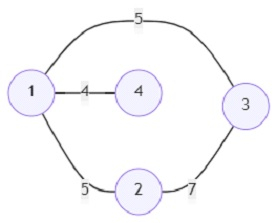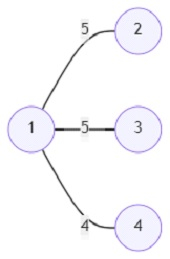# Program to find out an MST using Prim's algorithm in Python

Suppose we are given a graph and asked to find out the 'Minimum Spanning Tree' (MST) from that graph. An MST of a graph is a subset of a weighted graph where all the vertices are present and connected, and there exists no cycle in the subset. MST is called minimum because the total edge weight of the MST is the minimum possible from the graph. So, here we use Prim's MST algorithm and find out the total edge weight of the MST from a given graph.

So, if the input is like, number of vertices (n) is 4, and start vertex (s) = 3, then the output will be 14.

The MST from this graph will be this −The total edge weight of this MST is 14.

To solve this, we will follow these steps −

• Define a function mst_find() . This will take G, s
• distance := a new list of size G initialized with value negative infinity
• distance[s] := 0
• itr := a new list of size G initialized with value False
• c := 0
• while True, do
• min_weight := infinity
• m_idx := -1
• for i in range 0 to size of G, do
• if itr[i] is same as False, then
• if distance[i] < min_weight, then
• min_weight := distance[i]
• m_idx := i
• if m_idx is same as -1, then
• come out from the loop
• c := c + min_weight
• itr[m_idx] := True
• for each pair i, j in values of G[m_idx], do
• distance[i] := minimum of distance[i], j
• return c
• G := a new map containing n other maps
• for each item in edges, do
• u := item
• v := item
• w := item
• u := u - 1
• v := v - 1
• min_weight = min(G[u, v], w)
• G[u, v] := min_weight
• G[v, u] := min_weight
• return mst_find(G, s)

## Example

Let us see the following implementation to get better understanding −

def mst_find(G, s):
distance = [float("inf")] * len(G)
distance[s] = 0
itr = [False] * len(G)
c = 0
while True:
min_weight = float("inf")
m_idx = -1
for i in range(len(G)):
if itr[i] == False:
if distance[i] < min_weight:
min_weight = distance[i]
m_idx = i
if m_idx == -1:
break
c += min_weight
itr[m_idx] = True
for i, j in G[m_idx].items():
distance[i] = min(distance[i], j)
return c

def solve(n, edges, s):
G = {i: {} for i in range(n)}
for item in edges:
u = item
v = item
w = item
u -= 1
v -= 1
try:
min_weight = min(G[u][v], w)
G[u][v] = min_weight
G[v][u] = min_weight
except KeyError:
G[u][v] = w
G[v][u] = w
return mst_find(G, s)

print(solve(4, [(1, 2, 5), (1, 3, 5), (2, 3, 7), (1, 4, 4)], 3))


## Input

4, [(1, 2, 5), (1, 3, 5), (2, 3, 7), (1, 4, 4)], 3

## Output

14